# JP4878062B2 - Pitching control apparatus and control method for automobile - Google Patents

## Info

Publication number
JP4878062B2
JP4878062B2 JP2009503979A JP2009503979A JP4878062B2 JP 4878062 B2 JP4878062 B2 JP 4878062B2 JP 2009503979 A JP2009503979 A JP 2009503979A JP 2009503979 A JP2009503979 A JP 2009503979A JP 4878062 B2 JP4878062 B2 JP 4878062B2
Authority
JP
Japan
Prior art keywords
motor
wheel
vehicle
acceleration
pitch angle
Prior art date
Legal status (The legal status is an assumption and is not a legal conclusion. Google has not performed a legal analysis and makes no representation as to the accuracy of the status listed.)
Expired - Fee Related
Application number
JP2009503979A
Other languages
Japanese (ja)
Other versions
JPWO2008111436A1 (en
Inventor

Original Assignee

Priority date (The priority date is an assumption and is not a legal conclusion. Google has not performed a legal analysis and makes no representation as to the accuracy of the date listed.)
Filing date
Publication date
Priority to JP2007054614 priority Critical
Priority to JP2007054614 priority
Priority to JP2007213184 priority
Priority to JP2007213184 priority
Application filed by 国立大学法人横浜国立大学 filed Critical 国立大学法人横浜国立大学
Priority to JP2009503979A priority patent/JP4878062B2/en
Priority to PCT/JP2008/053877 priority patent/WO2008111436A1/en
Publication of JPWO2008111436A1 publication Critical patent/JPWO2008111436A1/en
Application granted granted Critical
Publication of JP4878062B2 publication Critical patent/JP4878062B2/en
Application status is Expired - Fee Related legal-status Critical
Anticipated expiration legal-status Critical

## Images

•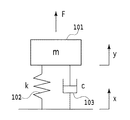•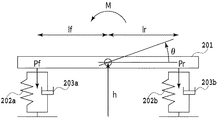•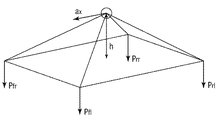•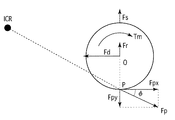•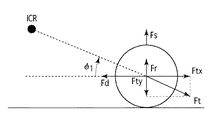•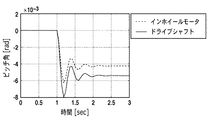•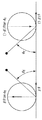•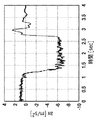•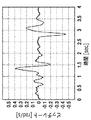•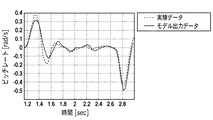•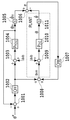•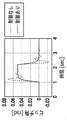•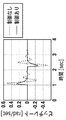•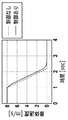•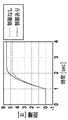•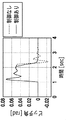•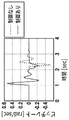•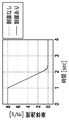•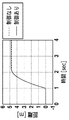•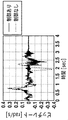•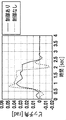•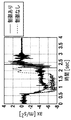•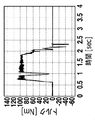•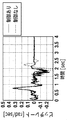•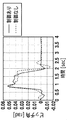•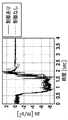•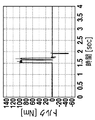••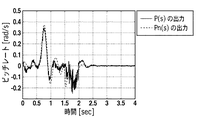•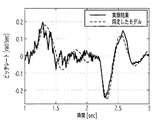•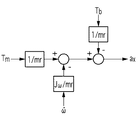•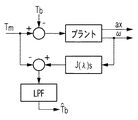•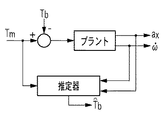•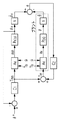•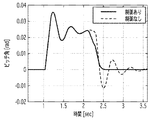•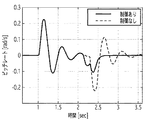•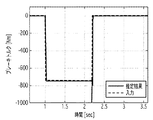•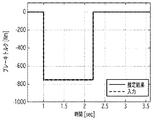•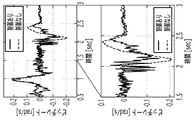•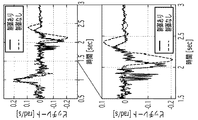•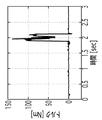•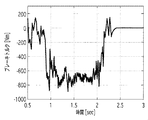•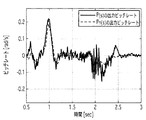•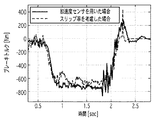•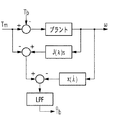•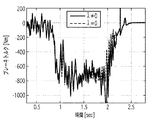•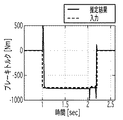•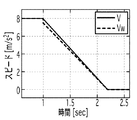•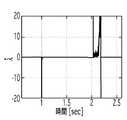•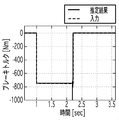•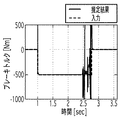•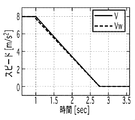•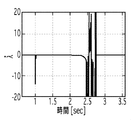•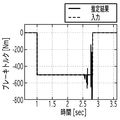•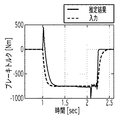•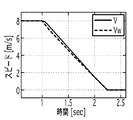•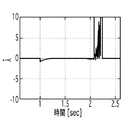•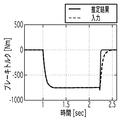•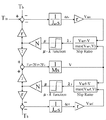•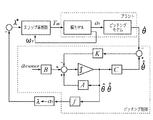•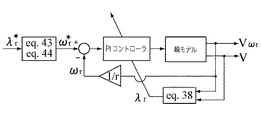•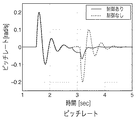•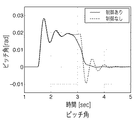•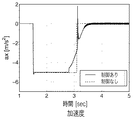•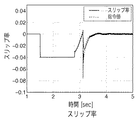•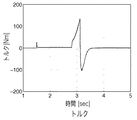•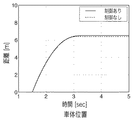•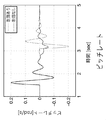•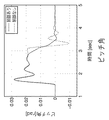•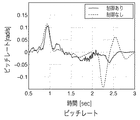•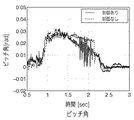•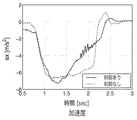•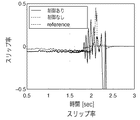•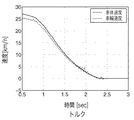•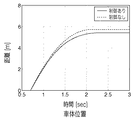## Classifications

• BPERFORMING OPERATIONS; TRANSPORTING
• B60VEHICLES IN GENERAL
• B60LPROPULSION OF ELECTRICALLY-PROPELLED VEHICLES; SUPPLYING ELECTRIC POWER FOR AUXILIARY EQUIPMENT OF ELECTRICALLY-PROPELLED VEHICLES; ELECTRODYNAMIC BRAKE SYSTEMS FOR VEHICLES IN GENERAL; MAGNETIC SUSPENSION OR LEVITATION FOR VEHICLES; MONITORING OPERATING VARIABLES OF ELECTRICALLY-PROPELLED VEHICLES; ELECTRIC SAFETY DEVICES FOR ELECTRICALLY-PROPELLED VEHICLES
• B60L15/00Methods, circuits, or devices for controlling the traction-motor speed of electrically-propelled vehicles
• B60L15/20Methods, circuits, or devices for controlling the traction-motor speed of electrically-propelled vehicles for control of the vehicle or its driving motor to achieve a desired performance, e.g. speed, torque, programmed variation of speed
• B60L15/2036Electric differentials, e.g. for supporting steering vehicles
• YGENERAL TAGGING OF NEW TECHNOLOGICAL DEVELOPMENTS; GENERAL TAGGING OF CROSS-SECTIONAL TECHNOLOGIES SPANNING OVER SEVERAL SECTIONS OF THE IPC; TECHNICAL SUBJECTS COVERED BY FORMER USPC CROSS-REFERENCE ART COLLECTIONS [XRACs] AND DIGESTS
• Y02TECHNOLOGIES OR APPLICATIONS FOR MITIGATION OR ADAPTATION AGAINST CLIMATE CHANGE
• Y02TCLIMATE CHANGE MITIGATION TECHNOLOGIES RELATED TO TRANSPORTATION
• Y02T10/00Road transport of goods or passengers
• Y02T10/60Other road transportation technologies with climate change mitigation effect
• Y02T10/64Electric machine technologies for applications in electromobilty
• Y02T10/642Control strategies of electric machines for automotive applications
• Y02T10/645Control strategies for dc machines
• YGENERAL TAGGING OF NEW TECHNOLOGICAL DEVELOPMENTS; GENERAL TAGGING OF CROSS-SECTIONAL TECHNOLOGIES SPANNING OVER SEVERAL SECTIONS OF THE IPC; TECHNICAL SUBJECTS COVERED BY FORMER USPC CROSS-REFERENCE ART COLLECTIONS [XRACs] AND DIGESTS
• Y02TECHNOLOGIES OR APPLICATIONS FOR MITIGATION OR ADAPTATION AGAINST CLIMATE CHANGE
• Y02TCLIMATE CHANGE MITIGATION TECHNOLOGIES RELATED TO TRANSPORTATION
• Y02T10/00Road transport of goods or passengers
• Y02T10/60Other road transportation technologies with climate change mitigation effect
• Y02T10/72Electric energy management in electromobility
• Y02T10/7258Optimisation of vehicle performance
• Y02T10/7275Desired performance achievement

## Description

The present invention relates to an automobile pitching control apparatus and control method, and more particularly to an automobile pitching control apparatus and control method for controlling pitching by motor torque control.

In terms of energy and environmental issues, electric vehicles are very superior to internal combustion engine vehicles and are attracting attention. However, an electric vehicle that uses a motor as a driving force has a good response from the torque command value to the generated torque, which is a feature of the motor, and can accurately grasp the generated torque value of the motor by measuring the motor current. Since the motor is compact, it has a great advantage in that it can be distributed in each tire wheel (see Non-Patent Documents 1 and 2). Focusing on the characteristics of this motor, research on vehicle control unique to electric vehicles has been conducted (see Non-Patent Document 3).

The vehicle motion includes a pitching motion that greatly affects the ride comfort. Pitching is a movement around the center of gravity of the vehicle that occurs when a driving force or braking force is applied while the vehicle is running on a straight line, and a moment around the center of gravity (y-axis) of the vehicle is applied at the same time. is there. The present invention controls the pitching motion.

As a pitching control method that has been proposed so far, feed-forward control is performed in which a predetermined correction torque is applied to the measured pitching amount in a direction in which the pitching amount is suppressed, and the direction of the correction torque is changed every time the polarity changes. (See Patent Documents 1 and 2). Similarly, there is a pitching control method in which state feedback is performed on a model using a detailed sprung vibration model, although it is feedforward control (Patent Document 3).

However, there has been no model that precisely analyzes the pitching motion of the vehicle, and there has been no pitching control device that uses the high-speed response of the motor, which is a feature of an electric vehicle. Actually, the acceleration a xm due to the motor torque depends not only on the torque command value but also on the road surface condition. For this reason, there is a problem that an error of a xm is large and it is difficult to perform highly accurate pitching control. Furthermore, because the motor Hall sensor has a low resolution, it can be obtained by differentiating the wheel angular velocity ω with low accuracy when calculating the brake torque and nominal acceleration.

(Ωdot) There is a problem that a large noise is applied to the wheel angular acceleration and the accuracy of the pitching control is lowered.

The present invention has been made in view of such problems, and an object thereof is to provide an automotive pitching control device and a control method for controlling pitching motion by controlling torque of drive wheels. It is another object of the present invention to provide a highly accurate pitching control device and control method based on torque control of a motor based on a brake torque estimation method in consideration of a slip ratio. It is another object of the present invention to provide a highly accurate pitching control device and control method that considers road surface conditions without using wheel angular acceleration.

The present invention is a pitching control device, in a vehicle that drives a drive wheel with a motor torque, a load applied to each wheel of the vehicle when stationary, a load applied to each wheel of the vehicle during acceleration / deceleration by the motor, And an anti-dive force acting on the front wheel of the vehicle and a lift force acting on the rear wheel of the vehicle at the time of braking by the brake from a moment around the center of gravity of the vehicle based on a change in load applied to each wheel of the vehicle. a nominal pitch angle calculating means for calculating a nominal pitch angle of the motor torque calculating means for calculating a motor torque of the motor based on the nominal pitch angle, and a motor control means for controlling the motor using the motor torque It is characterized by having.

Another embodiment of the present invention, further comprising a feedback control means for compensating a difference between the actual pitch rate of the the nominal pitch rate derived from the nominal pitch angle calculated motor vehicle by the nominal pitch angle calculating means Features.

Further, one aspect of the present invention further includes a brake torque estimating unit that estimates a brake torque, wherein the nominal pitch angle calculating unit calculates a motor acceleration of the motor based on the brake torque.

Moreover, one aspect of the present invention is characterized in that the brake torque estimating means performs estimation in consideration of a slip ratio.

Moreover, one aspect of the present invention is characterized in that the brake torque estimating means performs estimation in consideration of a time change of the slip ratio.

Moreover, one aspect of the present invention further includes an acceleration sensor that measures the vehicle body acceleration of the automobile, and the brake torque estimating means performs estimation using the vehicle body acceleration .

Another aspect of the present invention is a pitching control device, in an automobile that drives driving wheels with motor torque, an acceleration sensor that measures an acceleration of a vehicle body of the automobile, the acceleration, and the acceleration of the automobile when stationary. The vehicle according to the load applied to each wheel, the load applied to each wheel of the vehicle during acceleration / deceleration by the motor, the anti-dive force acting on the front wheel of the vehicle and the anti-lift force acting on the rear wheel of the vehicle during braking by the brake A state variable including the nominal pitch angle is estimated by a state observer using a model for calculating a nominal pitch angle from a moment around the center of gravity of the vehicle based on a change in a load applied to each wheel of the vehicle, and the state variable is estimated State feedback control means using the value, and scan calculated by the state feedback control means. Tsu based on up rate, and wheel speed control means for calculating a motor torque of the motor, characterized in that a motor control means for controlling the motor using the motor torque.

Further, according to one aspect of the present invention, the wheel speed control means determines a control gain by a pole placement method considering only the inertia moment of the wheel, and the pole is constant with respect to the inertia moment of the wheel during acceleration / deceleration. The control gain is adjusted so that

Further, one aspect of the present invention is characterized in that the nominal pitch calculation means calculates the nominal pitch angle based on the formula (A).

(M: body weight, h: height from the ground contact surface to the center of gravity, l f : distance between the center of gravity and the front wheel shaft, l r : distance between the center of gravity and the rear wheel shaft, I: around the y axis of the vehicle body Moment of inertia, C: damper coefficient, K: spring constant, β: front wheel distribution of braking force, φ f : anti-dive force direction, φ r : anti-lift force direction)
In another aspect of the present invention, there is provided a pitching control method for a vehicle in which driving wheels are driven by a motor torque. A load applied to each wheel of the vehicle when stationary, a load of the vehicle during acceleration / deceleration by the motor, and the like. The center of gravity of the vehicle based on the load applied to each wheel and the change in the load applied to each wheel of the vehicle due to the anti-dive force acting on the front wheel of the vehicle and the anti-lift force acting on the rear wheel of the vehicle when braking by the brake a nominal pitch angle calculating step of calculating a nominal pitch angle of the drive wheel from the moment about the motor torque calculating a motor torque of the motor based on the nominal pitch angle, the motor using the motor torque And a motor control step for controlling.

Further, one aspect of the present invention further includes a feedback control step for compensating for a difference between the nominal pitch rate derived from the nominal pitch angle calculated in the nominal pitch angle calculation step and the actual pitch rate of the vehicle. And

Further, one aspect of the present invention further includes a brake torque estimating step of estimating a brake torque, and the nominal pitch angle calculating step calculates a motor acceleration of the motor based on the brake torque.

Further, one aspect of the present invention is characterized in that the brake torque estimating step performs estimation in consideration of a slip ratio.

Further, one aspect of the present invention is characterized in that the brake torque estimating step further performs estimation in consideration of a time change of the slip ratio.

Further, one aspect of the present invention further includes an acceleration measurement step of measuring the vehicle body acceleration of the automobile with an acceleration sensor, and the brake torque estimation step performs estimation using the vehicle body acceleration.

Another aspect of the present invention is a pitching control method, in an automobile in which driving wheels are driven by motor torque, an acceleration measuring step of measuring acceleration of the vehicle body by an acceleration sensor, and the acceleration, when stationary The load applied to each wheel of the vehicle, the load applied to each wheel of the vehicle during acceleration / deceleration by the motor, the anti-dive force acting on the front wheel of the vehicle and the anti-lift acting on the rear wheel of the vehicle during braking by the brake Estimating a state variable including the nominal pitch angle by a state observer using a model for calculating a nominal pitch angle from a moment around the center of gravity of the vehicle based on a change in load applied to each wheel of the vehicle by force, A state feedback control step using estimated values of the state variables, and the state feedback Based on the slip ratio calculated by the control step, and the wheel speed control step of calculating the motor torque of the motor, and having a motor control step of controlling the motor using the motor torque.

Further, according to one aspect of the present invention, the wheel speed control step determines a control gain by a pole placement method considering only the inertia moment of the wheel, and the pole is constant with respect to the inertia moment of the wheel during acceleration / deceleration. The control gain is adjusted so that

One embodiment of the present invention is characterized in that the nominal pitch angle calculation step calculates the pitch angle based on the formula (A).

(M: body weight, h: height from the ground contact surface to the center of gravity of the vehicle body, l f : distance between the center of gravity and the front wheel shaft, l r : distance between the center of gravity and the rear wheel shaft, I: y of the vehicle body Moment of inertia about the axis, C: damper coefficient, K: spring constant, β: front wheel distribution of brake force, φ f : angle between the ground plane and anti-dive force direction, φ r : ground plane and anti-lift force direction Angle between)

According to the present invention, it is possible to control the pitching motion by controlling the torque of the drive wheels. In addition, in a motor vehicle powered by a motor, high-precision pitching control can be performed by motor torque control based on a brake torque estimation method in consideration of a slip ratio. In addition, highly accurate pitching control can be performed in consideration of road surface conditions without using wheel angular acceleration.

The embodiment of the present invention described below is described as being mounted on an electronic control unit (hereinafter referred to as “ECU”) of an automobile that drives driving wheels with the torque of a motor. The current output from the power supply is supplied to the motor via an inverter, and the motor is electrically connected to the ECU as the control means via the inverter. That is, the output of the motor is controlled by an inverter that controls the output current based on a command from the ECU. The ECU includes a CPU, a ROM, a RAM, an input / output port, a storage device, and the like. The ECU measures a torque generated by the motor, a position sensor mounted on the motor, and an acceleration that measures acceleration generated in the vehicle body. Sensors and the like can be electrically connected to each other.

[Embodiment 1]
1. Overview of Embodiment 1 FIG. 10 shows a block diagram of a control system of a pitching control device according to Embodiment 1 of the present invention. As will be described later, the nominal plant P n (s) has an anti-dive force and an anti-lift force in addition to the load applied to each wheel at rest and the load applied to each wheel during acceleration / deceleration due to the torque of the motor. Is modeled so as to function as a nominal pitch angle calculating means for calculating the nominal pitch angle θ n from the moment M around the center of gravity of the vehicle based on the change in the load of each wheel in consideration of the above.

If you enter the acceleration a xb by the braking force to the nominal plant P n (s), the feedforward controller based on the nominal pitch angle theta n output from the pitch angle command value theta * a nominal plant P n (s) C FF Outputs the motor acceleration a xm that is ideally controlled. The acceleration a xb by the braking force is obtained by subtracting the acceleration a xm by the motor calculated based on the current value to the motor from the acceleration a x of the vehicle body measured by the acceleration sensor.

When the acceleration a xm by the motor and the acceleration a xb by the braking force output from the feedforward controller C FF are input to the actual plant P (s), the actual pitch angle θ is output from the actual plant P (s). Actual pitch rate obtained by differentiating actual pitch angle θ

The feedback controller C FB compensates for the motor acceleration a xm based on the difference (e ) between (θdot) and the nominal pitch rate θ n dot obtained by differentiating the nominal pitch angle θ n .

Since feedback control is performed in this way, it is less susceptible to modeling errors.

Hereinafter, the pitching motion model will be described in detail. Since the pitching motion is a rotational motion around an axis perpendicular to the traveling direction and also perpendicular to the road surface (y-axis when the x-axis is the traveling direction in a plane parallel to the road surface), acceleration is considered. A plant model is created by determining the transfer functions of the front and rear wheels. At that time, the difference in pitching effect between in-wheel drive and non-in-wheel drive is described, and the anti-dive and anti-lift effects due to braking force are also described. In the present invention, identification based on the experimental data is performed on the created pitching motion model, and pitching is controlled based on the identification model, so the identification method and experimental results are also described below.

2. Modeling of pitching motion and derivation of transfer function 2.1 Half car model Pitching is a change in the posture of the vehicle body, and can be approximated by a model that considers only the vehicle body (on the spring). Moreover, since it is a motion in the front-rear direction, it can be considered as a two-wheel model (half car model). Therefore, it can be represented by a half car model as shown in FIG. 2 (see Non-Patent Document 4).
The transfer function of the one-degree-of-freedom model as shown in FIG.

It can be expressed as. The one-degree-of-freedom model considers vertical motion, but the half-car model considers a rotating system, so the vehicle body weight m can be replaced by the vehicle body inertia moment I, and the spring constant k and the damper coefficient c are C in the half-car model. , K, the half-car model can be considered equivalent to a one-degree-of-freedom model. Therefore, the transfer function of the half car model can be expressed as follows (see Non-Patent Document 4).

Where I [kgm2] is the moment of inertia about the y-axis of the vehicle body, C [Ns / m] is the damper coefficient, K [N / m] is the spring constant, θ [rad] is the pitch angle, P f , P r [ N] is a load applied to the front and rear wheels during acceleration.

2.2 Load change due to longitudinal acceleration Pitch motion occurs when the vehicle accelerates or decelerates. Therefore, in order to consider the acceleration, the change in load due to the longitudinal acceleration will be considered. Load of each wheel during steady movement when the longitudinal acceleration a x is given by the following equation (see Non-Patent Document 5).

However, P fr , P fl , P rr , P rl [N] are the loads of each wheel, N f , N r [N] are the loads of the front and rear wheels when stationary, m [kg] is the weight of the vehicle body, h [M] is the height of the center of gravity, and l f and l r [m] are the distances between the center of gravity and the front and rear wheel shafts.

Consider the load on the front and rear wheels to consider a half car model. At this time, the following equation is obtained.

P f and P r are loads applied to the front and rear wheels, respectively. Here, the moment of force M [Nm] around the center of gravity in FIG.

Therefore, when substituting the formulas (7) and (8) into (9) into the formula (2), it is expressed as follows.

The first term on the right side of the above equation is 0 because it is a moment at rest. From this, it can be written as:

From the above, the transfer function from the acceleration a x [m / s2] to the pitch angle θ could be expressed.

3. Pitching suppression effect at start-up <3.1> The case of in-wheel drive is shown in FIG. In the case of in-wheel drive, since the motor is under the suspension, the mechanism receives a force from above the suspension via the upper arm and the lower arm. With respect to this force, the rotational force of the motor acts as a balance force, and it is considered that the balance of the wheels is the ground contact surface. From this, it is considered that the operating point of the driving force is on the ground contact surface of the wheel (see Non-Patent Document 6). At this time, a force called F p acts on the ground contact surface and can be decomposed into F px and F py . Considering the balance of forces in the horizontal direction, F px = F d can be obtained. F d is a driving force. When considering the balance of force in the vertical direction, if Fr is considered to be the vertical load of the rear wheels (acceleration load + static load),

Thus, F d tanφ is a force that suppresses pitching, and Fs is a force that actually works.

3.2 Non-in-wheel type drive On the other hand, as shown in FIG. 5, in the case of non-in-wheel type drive (drive shaft drive), the motor is installed on the suspension. There is no need to support. That is, since no couple is generated under the suspension, the point of action of the force is concentrated at the center of the wheel (see Non-Patent Document 6). This can be expressed by the following equation as in the case of in-wheel drive.

Than this suppresses force F d tanφ1 pitching the case of non-wheel motor works but, phi> because it is φ 1 tanφ> tanφ 1, and the pitching suppression than non-wheel drive is more in-wheel motor drive It turns out that the effect is great.

3.3 Comparison of pitching effect by simulation For reference, a simulation is performed at the start in an open loop in each case of in-wheel type drive and drive shaft drive.

As will be described later in Chapter 5, the simulation was performed based on the pitching model obtained by the identification test. In this case, the acceleration is t = 1.0 s and the step input is a x = 1.0 m / s2, and the values of φ in the case of in-wheel drive and drive shaft drive are φ = 30 and 20 deg, respectively. The pitch angle is shown in FIG. From this figure, it can be seen that the pitching is smaller in the case of the in-wheel type drive.

4). Anti-dive and anti-lift geometry during braking When starting, the pitching effect of the in-wheel motor discussed in Chapter 3 can be taken into account, but during braking, the braking force works and the motor does not work. Consider anti-dive and anti-lift geometry with such a braking force (see Non-Patent Document 7).

During braking, braking force acts on the front and rear wheels. When the front wheel distribution of the brake force is β, when the total brake force acting on the front and rear wheels is F, the front wheel brake force is βF and the rear wheel brake force is (1−β) F. Since the brake torque is transmitted to the suspension through the brake unit, the virtual action point of force is considered to be on the ground surface as shown in FIG. 7, and the anti-dive force βFtanφ f is applied to the front wheel and the anti-lift force (1 -Β) Ftanφ r works.

Considering this, modeling is performed again. The load on the front and rear wheels

Next, the moment of force, because it is F = -ma x when you ignore the running resistance,

And the transfer function is

It becomes. The first term of the right-hand numerator is the term that appears due to the inertial force, and the second term is the term that appears due to the braking force acting on the contact surface. This time, the pitching control during braking is controlled based on this model, but because there are many unknown parameters, the above model is identified based on experimental data.

5. Parameter identification and experimental results Since the model parameters obtained up to the previous section are unknown, identification is necessary. Therefore, an identification experiment was conducted.

<5.1> Actual machine specifications The experimental machine uses a commercially available small electric vehicle EV-1 (Cimotors Qi (QUINO)).

The motor is controlled using an inverter system manufactured in cooperation with Myway Giken. Further, since the resolution of the Hall sensor of the motor is low, the position angle is vector-controlled by linear interpolation. The sampling period is 10 kHz.

The <5 · 2> parameter identification experiment conditions, and it shall provide an acceleration a x in steps, by placing those fixed to the back of the brake pedal in time, and so the braking force becomes constant.

As for the pitch rate θdot, since this laboratory does not have a pitch angle sensor, an experiment was performed with a yaw sensor attached to the y-axis. The experimental results (acceleration, pitch rate) at that time are shown in FIGS. 8A and 8B. From this experimental result, the input was identified as acceleration a x and the output as pitch rate θdot.

As an identification method, in this paper, the parameters of the transfer function were obtained so that the outputs corresponding to the inputs in FIGS. 8A and 8B fit in the time domain. The transfer function is shown below.

At this time, the natural angular frequency ω n = 16.7 rad / s and the attenuation constant ζ = 0.23.

Moreover, the verification result in this identified model is shown in FIG. From this figure, it can be seen that the experimental output and the identified model output data have fairly close waveforms.

Expression (18) is a transfer function of acceleration input pitch rate output, and the pitch rate is obtained by differentiating the pitch angle. Therefore, the transfer function of acceleration input pitch angle output is expressed by the following expression.

In the first embodiment, the pitching used in this model is controlled.

6). Simulation Results Assuming that F xb is a driving force by a brake force and F xm is a driving force by a motor, it is assumed that the driving force dimension can be expressed by the following expression.

By dividing both sides of formula (20) in m, from the equation F x = ma x,

Can be expressed as a xb is the acceleration due to the braking force, and a xm is the acceleration due to the motor. Based on this equation, a control system using a two-degree-of-freedom control system as shown in the block diagram of FIG. 10 is proposed. This control system is ideally realized by CFF having a sufficiently high pitch angle when the acceleration by the braking force is given as an input to the nominal plant P n (s) having the transfer function of the equation (19) identified in Chapter 5. Axm to be controlled automatically . This is applied to the actual plant P (s) as motor torque. When the actual plant is the same as the nominal plant, pitching can be ideally suppressed by this motor torque. If there is a modeling error, and the actual pitch rate, which is the output from the actual plant, the difference between the nominal pitch rate determined by quasi differentiated by the nominal pitch angle high pass filter is the output of the nominal plant, the feedback controller C The motor torque compensated by FB is applied to the actual plant. Thereby, it is possible to have high controllability even when there is a modeling error. This control system simulates pitching control. At this time, the constants used were r = 0.22 m, m = 420 kg, and the nominal plant parameters used were the values identified in Chapter 5.

As a simulation condition, a vehicle traveling at a constant speed is suddenly braked after t = 1.0 s, and a pitch angle, a pitch rate, a vehicle body speed, and a vehicle body position until stopping are observed. Also, in order to have a modeling error, the spring and damper coefficients of P n (s) and P (s) are C n = 1250 Ns / m, K n = 45500 N / m, C = 500 Ns / m, K = 45000 N, respectively. / M. CFF was designed as a PD controller, and CFB was designed as a PI controller by the pole placement method.

<6.1> Simulation 1
First, FIGS. 11A to 11D show the results of simulations comparing the case without open loop control and the case with control. At this time, the values of the closed loop poles of C FF and C FB were 17 rad / sec and 10 rad / sec, respectively. 11A and 11B that the pitch angle and the pitch rate are well suppressed. However, it can be seen from FIG. 11D that the distance until the vehicle body stops has increased considerably.

6.2 Simulation 2
In order to solve the problem of the simulation 1, a simulation was performed in which control was performed when the vehicle body speed V became lower than 1.0 m / s. The result is FIG. 12A-FIG. 12D. At this time, the values of the closed loop poles of C FF and C FB were 23 rad / sec and 10 rad / sec, respectively. It can be seen from FIG. 12D that the distance to stop is almost the same as in the case without control. However, since the control is started when the vehicle body speed V becomes lower than 1 m / s, the pitch rate can be suppressed only after 2.04 s.

7). Actual machine verification The simulation results of Chapter 6 were verified. As conditions in the experiment, as in the identification experiment in Chapter 5, sudden braking is applied from a constant speed on the dry road. At that time, the braking force was made constant. The acceleration a xb due to the braking force that is input to the nominal plant is assumed to be obtained by subtracting the acceleration a xm from the motor that is input to the actual plant from the output a x from the acceleration sensor, from Equation (21). The values of the closed loop poles of C FF and C FB were the same as in the simulation. The case with control at that time and the case without control will be compared. Measurement data includes acceleration a x ,

, Wheel speed V ω and torque T.

<7.1> Experiment 1
First, an experiment on simulation 1 in Chapter 6 was performed. The experimental results at this time are shown in FIGS. 13A to 13D. FIG. 13A, FIG. 13B, and FIG. 13C are views from the angular velocity sensor with and without control, respectively.

If its pitch angle pitch rate was determined by quasi differentiated by the high-pass filter theta, also a result of comparing the acceleration a x at that time, FIG. 13D is the actual torque T of the controlled case.

In the experimental result of FIG. 13A, it can be seen that the pitch rate is suppressed in the case of control as compared to the case of no control. Also, in FIG. 13B, it can be seen that the pitch angle is also controlled with control compared to without control.

From FIG. 13C, it can be seen that the acceleration is suppressed with the control as compared with the case without the control, and the distance to stop is extended.

FIG. 15 compares the output pitch rates from P (s) and P n (s), but the output of P (s) does not completely follow the output of P n (s). .

<7.2> Experiment 2
Next, actual machine verification in simulation 2 was performed. Since the experiment was conducted on a dry road, the control was started when the wheel speed reached about 3 km / h, assuming that the slip was very small. The experimental results at this time are shown in FIGS. 14A to 14D. As in Experiment 1, the pitch rate, pitch angle, acceleration, and actual torque are shown.

Also in this case, it can be seen from FIGS. 14A and 14B that both the pitch rate and the pitch angle are suppressed as in the simulation results.

Further, from FIG. 14C, since control is applied immediately before stopping, the acceleration decreases only immediately before stopping. From this, it can be seen that the distance to stop does not extend much compared to the case of Experiment 1.

FIG. 16 compares the output pitch rates of P (s) and P n (s) in the same manner as in FIG. 15, but a slight error occurs although the waveforms are close to each other. This is also considered to be the same as the cause in FIG .

[Embodiment 2]
In the first embodiment, the acceleration a xb due to the braking force is derived from the acceleration a x of the vehicle body measured by the acceleration sensor and the acceleration a xm by the motor calculated based on the current value output to the motor. However, since the motor acceleration axm is more strictly affected by the road surface state, it is desirable to derive the acceleration in consideration of the road surface state. Hence, the second embodiment derives the vehicle body acceleration a x from the equation of motion of the vehicle.

FIG. 21 shows a block diagram of a control system using brake torque estimation of the pitching control device according to the second embodiment of the present invention. As in the first embodiment, the nominal plant P n (s) calculates the pitch angle θ n from the moment M around the center of gravity of the vehicle based on the change in the load of each wheel considering the anti-dive force and anti-lift force. Modeled to be. A x in FIG. 21 is the longitudinal acceleration estimator of FIG. Also in the A x, the estimated value of the braking torque T b from estimator using the vehicle acceleration a x by the acceleration sensor shown in the estimator, or 20 considering the slip ratio shown in FIG. 19 are input. ωdot is a value obtained by differentiating the wheel speed ω with respect to time.

This control system derives the nominal acceleration a xn from the brake torque T b calculated by the brake torque estimator, and when the nominal acceleration a xn is given to the nominal plant P n (s), it is idealized by the feedforward controller C 1 . The nominally controlled nominal motor torque T mn is obtained. By applying this to the actual plant P (s) as the motor torque, when the actual plant is the same as the nominal plant, the pitching can be suppressed by the motor torque T mn .

If there is a modeling error in the nominal plant P n (s), is the differential value of the nominal pitch angle theta n is the output of the actual pitch rate θdot which is the output from the actual plant, the nominal plant P n (s) The motor torque T m compensated for the difference in the nominal pitch rate θ n dot by the feedback controller C 2 is combined with the nominal motor torque T mn and applied to the actual plant. This makes it possible to have high controllability even when there is a modeling error.

The second embodiment can perform more precise pitching control than the first embodiment by performing the pitching control using the brake torque estimated in consideration of the slip ratio.

Based on the contents of Chapters 1 to 4, Embodiment 2 will be described in detail below.

9. Parameter Identification Similarly to the first embodiment, the second embodiment performs control based on models in which the moment of force and the transfer function of the acceleration input pitch rate output are given by the equations (16) and (17), respectively. Since the experimental vehicle different from the first embodiment is used in the second embodiment, unknown parameters are identified in the same manner as in the first embodiment based on the following experiment.

<9.1> Actual machine specifications The experimental machine uses a commercially available small electric vehicle EV-3 (COMS LONG BASIC). The use of the inverter / motor is the same as that of the experimental vehicle EV-1 used in the first embodiment.

<9.2> Parameter Identification The experiment was performed under the same conditions as in the first embodiment. FIG. 17 shows a result of comparison between the experimental pitch rate and the output pitch rate when the acceleration at that time is input to the identified model. As an identification method, in the second embodiment, the parameters of the transfer function are obtained so that the output corresponding to the input of acceleration is fitted in the time domain. The transfer function is shown below.

At this time, the natural angular frequency ω n = 14.3 rad / s and the attenuation constant ζ = 0.22. From FIG. 17, it can be seen that the experimental result shows that although the pitch rate value is small, noise is added, but the waveform is close.

Since Expression (22) is a transfer function of acceleration input pitch rate output, and the pitch rate is obtained by differentiating the pitch angle, the transfer function of acceleration input pitch angle output is obtained by integrating Expression (22).

10. Calculation Method of Nominal Acceleration <10.1> Nominal Acceleration In the first embodiment described above, the acceleration serving as the plant input is obtained as the sum of the acceleration due to the motor and the acceleration due to the braking force, as shown in equation (21).
However, in actuality, a xm is determined by the acceleration by the motor and the road surface condition. Therefore, the present invention proposes a method for calculating acceleration in consideration of road surface conditions.
First, the equation of motion of the vehicle is shown below.

Each variable includes a motor rotational speed ω [rad / s], a vehicle body speed V [m / s], a wheel speed V ω [m / s], a motor torque T m [Nm], a brake torque T b [Nm], Driving force F d [N]. The constants are a vehicle weight m [kg], a tire radius r [m], and a wheel rotating portion moment of inertia J ω [Nms 2 ].
When a x is obtained from equation (23), the following equation is obtained.

FIG. 18 is a block diagram showing a longitudinal acceleration estimator that estimates longitudinal acceleration from motor torque, brake torque, and wheel angular acceleration. Thereby, the acceleration which considered the road surface state can be calculated | required. However, in (26), the motor torque the T m can be measured, can not be measured wheel angular acceleration ωdot braking torque T b, it is necessary to separately estimate.

<10.2> Brake torque estimation In the second embodiment, the following two methods are proposed as a brake torque estimation method.

<10.2.1> Estimating considering slip ratio A brake torque estimation method considering the slip ratio is proposed.

The slip ratio is expressed as follows.

When F d and V are eliminated from the slip ratio equation (27) and equations (23) to (25) to obtain the brake torque T b , the equation (28) is obtained when V> Vω as shown below. , V ω > V, it is expressed as in equation (29).

Assuming that the slip ratio variation is minute and approximating λdot = 0, the equations (28) and (29) can be summarized as the following equations (30) and (31).

FIG. 19 is a block diagram showing a brake torque estimator considering the slip ratio. 19, when J (λ) = J brake ( λ) of V> V omega, when J (lambda) of the V ω> V = a J acc (λ). If the slip ratio can be measured from the equations (30) and (31), the brake torque T b can be estimated as shown in FIG. 19 by inputting the measured motor torque T m and wheel angular velocity ω. .

It shows the estimation of the braking torque T b using the estimated acceleration sensor using a <10, 2, 2> acceleration sensor. From the equations (23) and (24), the brake torque can be obtained as follows.

FIG. 20 is a block diagram showing a brake torque estimator using an acceleration sensor. If it is possible to correct the influence of gravity from the acceleration sensor during the pitching, the measured motor torque T m, the wheel angular acceleration Omegadot, braking torque T b as shown in FIG. 20 by entering the value of the vehicle body acceleration a x Can be estimated.

11. Two-degree-of-freedom control FIG. 21 is a block diagram showing a control system of a two-degree-of-freedom control system of the pitching control device according to one embodiment of the present invention. A x in this figure is the longitudinal acceleration estimator in FIG. Additional A x is 19 or estimator from the brake torque T b in FIG. 20, the motor torque T m, the wheel angular acceleration ωdot is input. In this control system, when a nominal acceleration a xn using an estimated brake torque value is given to the nominal plant P n (s) having the transfer function identified in Chapter 2, C 1 having a sufficiently high pitch angle is obtained. The nominal motor torque T mn is obtained by ideally controlling by This is applied to the actual plant P (s) as motor torque. When the actual plant is the same as the nominal plant, the pitching can be suppressed by this nominal motor torque T mn .

If there is a modeling error, the motor torque compensated by the feedback controller C 2 for the difference between the actual pitch rate output from the actual plant and the nominal pitch rate that is the differential value of the nominal pitch angle output from the nominal plant. the T m is applied to the actual plant. This makes it possible to have high controllability even when there is a modeling error.

12 Simulation Computer simulation was performed using the brake torque estimation method and pitching control method shown in the previous chapter. Parameters used in the simulation, the vehicle body weight m = 480 kg, the wheel radius r = 0.22 m, wheel axle moment of inertia J ω = 1.0 [Nms 2] , the nominal plant Pn (s) were identified model, the actual plant P (s) has a spring and a damper coefficient of C = 2450 Ns / m and K = 66000 N / m, respectively, in order to have a modeling error. Regarding the controller, C 1 was designed as a PD controller and C 2 was designed as a PI controller by the pole placement method, and the values of the respective closed-loop poles were 21 rad / s and 10 rad / s.

As a simulation condition, it is assumed that while traveling at a constant speed of 8.0 m / s on a high μ road, the vehicle is decelerated by applying a brake torque of 750 Nm and the vehicle stops. In addition, since there is a problem that the braking distance is extended when the control is always applied, the control is started when the speed is reduced, and this time the vehicle speed becomes 1.0 m / s or less. It is assumed that control starts to be applied from time.

22A and 22B show pitch angles and pitch rates with and without pitching control according to an embodiment of the present invention. FIG. 23A and FIG. 23B show the estimated brake torque value when the slip ratio is considered and the estimated value when the acceleration sensor is used.

22A and 22B show that both the pitch angle and the pitch rate are better suppressed with control according to the second embodiment than with no control. FIG. 23A shows the estimated value of the brake torque in consideration of the slip ratio at that time, and shows that it can be estimated with high accuracy. The waveform is slightly disturbed just before stopping, but this is thought to be due to the fact that the wheel speed and the vehicle body speed are reversed just before stopping, causing chattering. However, as FIG. 22A and FIG. 22B show, Embodiment 2 can control pitching with sufficient accuracy. Further, FIG. 23B shows the estimated brake torque value of the estimation method using the acceleration sensor. In this case, it shows that the estimation is better, and FIG. 22B shows that the accuracy of the pitching control is also increased. Show.

13. Actual machine verification Next, we conducted experiments using actual machines. The parameters used in the experiment were the vehicle body weight m = 480 kg, the wheel radius r = 0.22 m, and the nominal plant parameters used were the identified values. Regarding the controller, C 1 was designed as a PD controller, and C 2 was designed as a PI controller by the pole placement method. In this experiment, the value of each closed-loop pole was set to 21 rad / s and 10 rad / s as in the simulation.

As described above, there is a problem that the braking distance increases if the control is always applied. Therefore, the control is started when the speed is reduced. In this case, the vehicle is decelerated by braking while traveling at a constant speed of about 28 km / h, and control is started when the vehicle speed becomes 4.5 km / h or less.

Further, in this embodiment, the slip ratio is obtained by detecting the vehicle speed from the front wheel and the wheel speed from the rear wheel for simplification, but is obtained by estimating the slip ratio (see Non-Patent Document 8). A thing may be used.

Hereinafter, experimental results of pitching control using a brake torque estimation method considering a slip ratio and a brake torque estimation method using an acceleration sensor are shown. FIG. 24A shows the pitch rate with and without control using the estimation in consideration of the slip ratio according to one embodiment of the present invention, and FIG. 24B uses the acceleration sensor according to one embodiment of the present invention. The pitch rate with and without control using the estimated estimation is shown. Table 1 shows the peak-to-peak value of the pitch rate immediately before stopping (around 2 to 2.5 seconds).

From FIG. 24A, FIG. 24B, and Table 1, it can be seen that the pitch rate is better suppressed when the control is applied than when the control is not performed.

In the experiment, a stopper is placed on the back of the brake pedal so that the braking force is constant in all experiments. However, since the brake pedal is depressed with a person's foot, the braking force is exactly the same every time. However, the peak-to-peak values are not always the same, but the reference values are as shown in Table 1. If the maximum torque of the vehicle is increased, the pitch rate can be further suppressed.

Next, FIG. 25 shows an estimated value of the brake torque when the slip ratio is taken into consideration. FIG. 26 shows output pitch rates of the actual plant P (s) and the nominal plant Pn (s). The waveforms of the output pitch rates of the actual plant P (s) and the nominal plant Pn (s) coincide with each other when no control is applied. This indicates that the estimated value of the brake torque is a reasonable value.

FIG. 27 shows an estimated value of the brake torque using the acceleration sensor and an estimated value of the brake torque when the slip ratio is taken into consideration. Although the estimated value of the brake torque using the acceleration sensor is somewhat different from the estimated value of the brake torque considering the slip ratio, simple estimation of the brake torque is possible by using the acceleration sensor.

14 When the slip rate time change λdot is taken into consideration Until the previous chapter, the brake torque estimation method taking the slip rate into consideration assumed that the slip rate time change λdot was sufficiently small and λdot = 0 was estimated. Consider an estimation taking into account the term of the rate change λdot. FIG. 28 shows a block diagram for estimating the brake torque in consideration of the change λdot of the slip ratio. In FIG. 28, when V> , J (λ) = J brake (λ),

When V ω > V, J (λ) = J acc (λ),

It becomes.

FIG. 29 shows experimental results when estimation is performed with λdot ≠ 0 in which the slip ratio changes with time, and experimental results when estimation is performed with λdot = 0 where the slip ratio is constant. The experimental environment is the same as in the previous chapter. However, since there is not much difference from FIG. 29, verification by simulation was performed. The simulation conditions are the same as in Chapter 12. FIGS. 30A to 30D and FIGS. 31A to 31D show simulation results when brake torques 750 Nm and 500 Nm are applied on a high μ road, respectively. FIGS. 30A and 31A show the estimated brake torque values when λdot is taken into account, FIGS. 30B and 31B show the wheel speeds and vehicle body speeds in FIGS. 30A and 31A, and FIGS. 30C and 31C show FIGS. Λdot at 31A is shown, and FIGS. 30D and 31D show the estimated brake torque values when λdot = 0 and the slip ratio is constant.

30A to 30D, when the estimation is performed in consideration of the time variation λdot of the slip ratio, the estimated value of the brake torque is more violent than when the estimation is performed by approximating λdot = 0 with the constant slip ratio. You can see that This is considered to be due to the time change λdot of the slip ratio in FIG. 30B. 31A to 31D show that when the input brake torque is lowered to 500 Nm, the waveform of the estimated value is disturbed due to the influence of the time change λdot of the slip ratio.

Next, FIGS. 32A to 32D show simulation results in the case where a brake torque 750 Nm that gently rises and falls on a high μ road is applied. Similar to FIGS. 30A to 30D and FIGS. 31A to 31D, FIG. 32A is an estimated brake torque value when λdot is considered, FIG. 32B is a wheel speed / vehicle speed at the time of FIG. 32A, and FIG. FIG. 32D shows the estimated brake torque when the slip ratio is constant and λdot = 0. Since the brake torque is related to the amount of depression of the person's brake pedal, it is considered that the brake torque is not strictly given stepwise. Therefore, the simulation is performed by giving the stepped brake torque that has passed through a low-pass filter as input. It was. The cut-off of the low-pass filter was 20 rad / s.

Since the time variation λdot of the slip ratio in FIG. 32C is smaller than that without passing through the low-pass filter, the estimated value of the brake torque in FIG. 32A is also slightly improved. The reason why the estimated value becomes 0 earlier than the input value around t = 2.25 sec is that the vehicle has already stopped at that time.

[Embodiment 3]
FIG. 34 is a block diagram showing an overall control system of the pitching control device according to the third embodiment of the present invention. In the third embodiment, the wheel motion and the pitching motion are controlled separately by separating the slip ratio control system and the pitching control system. By doing so, the wheel angular velocity ω and the wheel angular acceleration ωdot that are easy to ride on with large noise used in the second embodiment are not used for pitching control.

As in the first and second embodiments, the pitching control system uses a model that calculates the pitch angle θ from the moment M around the center of gravity of the vehicle based on the change in the load on each wheel in consideration of the anti-dive force and anti-lift force. The state variable including the pitch angle is estimated by the state observer, and the state feedback control using the estimated value of the state variable is performed. The observer vector K and the feedback vector f are designed using the pole placement method.

Further, the slip ratio control system is the slip ratio control is used based on the wheel speed control, the slip ratio λ derived from the vehicle body acceleration a x generated by the pitching control system, wheel speed ω derived from the vehicle speed V Based on the above, an ideal motor torque Tm is generated. FIG. 35 shows a block diagram of a wheel speed control system of the pitching control device according to the third embodiment of the present invention. In the PI controller, the control gain is determined by the pole placement method for the inertia moment of the wheel, but the control gain is tuned online so as to keep the pole constant according to the change of the slip ratio.

Similarly to the second embodiment, the third embodiment will be described below in detail with respect to the third embodiment, along with the results of simulations and experiments performed using the model identified in the ninth chapter, based on the contents of the first to fourth chapters. explain.

15. Vehicle model The equation of motion around the rotation axis of the front and rear wheels is as follows.

Mechanical brakes generally work on four wheels. Braking torque T b of the four-wheel each wheel for the sake of simplicity in this embodiment are equal.

The equation of motion of the car body is as follows.

However, F d = 2F f + 2F r . The running resistance is ignored. Further, the slip ratio is expressed by Expression (27).

FIG. 33 shows a block diagram of a vehicle model in which these equations are combined, that is, a vehicle model used in the pitching control device according to the third embodiment of the present invention. The variables are the rotational angular speeds ω r and ω f [rad / s] of the motor, the vehicle speed V [m / s], the wheel speeds V ωr and V ωf [m / s], and the motor torque T m [Nm] of the driving wheels. ], The brake torque T b [Nm] of each wheel of the four wheels, the driving force F d [N] of the vehicle, and the driving forces F r and F f [N] of the front and rear wheels, respectively. The constants are the vehicle body weight m [kg], the tire radius r [m], and the wheel rotation portion inertia moments J ωr and J ωf [Nms2] of the front and rear wheels.

In the third embodiment, Magic Formula is adopted as the μ-λ curve representing the relationship between the friction coefficient between the tire and the road surface and the slip ratio.

16. Control System Design In the second embodiment, the wheel angular acceleration ωdot is used when determining the brake torque and the nominal acceleration. However, due to the low resolution of the motor Hall sensor, the wheel angular acceleration obtained by differentiating the rotational angular velocity of the motor is greatly affected by noise. In the third embodiment, therefore, a slip rate control system (see Non-Patent Document 8) based on wheel speed control is incorporated into the inner loop of the pitching control system, thereby controlling the pitching motion and the wheel motion separately. Thereby, it is possible to perform highly accurate control in consideration of the road surface state without using ωdot.

Further, while the feedforward-based pitching control system has been proposed in the first and second embodiments, the third embodiment uses state feedback control using an observer as the pitching control system. This further strengthens the influence of modeling errors and the like.

FIG. 34 is a block diagram showing an overall control system of the pitching control device according to the third embodiment of the present invention. Since the control input obtained from the pitching control system is an acceleration command, it is necessary to convert it to a slip rate dimension that is a command value of the slip rate control system. An expression showing the relationship between the slip ratio and the driving force is shown below.

D s indicates the driving stiffness, λ i is the slip ratio λ r , λ f , F i of the front and rear wheels, and driving forces F r , F f . From this equation (36) and equation (35), the command value for the rear wheel slip ratio can be obtained as follows. The pitching control system and the slip ratio control system used in the third embodiment will be described below.

From equation (37), the rear wheel slip ratio as a command value of the slip ratio control system can be obtained.

<16.1> Pitching control by state feedback The controlled object is expressed as follows from the equation (17).

Since the above system is observable,

It can be said that there exists a matrix K that determines the eigenvalues of. Therefore, the same-dimensional observer was designed. The observer gain vector obtained by pole arrangement is as follows. However, r 1 and r 2 are the poles of the observer.

State feedback control is performed using the state variables estimated by the observer shown above. As with the observer, the feedback matrix is obtained by pole arrangement. The obtained feedback matrix is as follows. However, w 1 and w 2 are the poles of the regulator.

<16.2> Slip ratio control based on wheel speed control The target wheel speed can be calculated from the target slip ratio λ * obtained from the pitching control system and the vehicle body speed. In this paper, it is assumed that the vehicle body speed can be detected from the sensor, but the vehicle body speed may be obtained by estimating the slip ratio (see Non-Patent Documents 8 and 10). At this time, it is conceivable that the wheel speed is higher than the vehicle body speed. Therefore, the case where the vehicle body speed is higher (formula (42)) and the case where the wheel speed is higher (formula (43)) is divided.

Thus, slip ratio control is realized by using rotational speed control including a speed loop outside a current control loop of a motor that is generally used.

FIG. 35 is a block diagram showing wheel speed control of the pitching control device according to the third embodiment of the present invention. In general, a speed controller uses a PI controller to determine a control gain by a pole placement method based on the following equation that considers only the moment of inertia of the wheel.

However, since the inertia moment of the entire vehicle body changes according to the change of the slip ratio, when the controller is designed only with the inertia moment of the wheel, the pole of the controller changes relatively according to the change of the slip ratio. Therefore, it is necessary to make the control gain variable so as to keep the pole constant.

Expressions (27) and (33)-(35) are summarized as follows for each case of V> V ω and V ω > V.

Expression (45) represents a case where V> V ω and Expression (46) represents a case where V ω > V. The control gain is tuned online so that the poles are constant with respect to these two formulas J break (λ) and J accel (λ).

17. Simulation A simulation was performed using the vehicle model in Chapter 15 and the control system in Chapter 16. In the simulation, it is assumed that a brake torque of 150 Nm is applied to each wheel while traveling on a high μ road (peak μ = 0.9) and the vehicle is stopped. At this time, in the first embodiment, if the pitching control is always applied, there is a problem that the braking distance is extended. Therefore, the slip ratio control system always operates with the command value λ r = −0.04, and the vehicle speed is 1.5 m. It is assumed that the command is switched to the slip ratio command value obtained from the pitching control system from the time of / s or less. In addition, the respective parameters were set to J ωr = 1.0 Nms2, J ωf = 0.5 Nms2, r = 0.22 m, m = 480 kg, and the driving stiffness was a constant value of D s = 15000.

First, FIGS. 36A to 36F show simulation results when the controlled object P (s) is equivalent to the identified model P n (s). At this time, the pole of the regulator was −3 rad / s, the pole of the observer was −10 rad / s, and the pole of the slip ratio control was −120 rad / s. 36A shows the pitch rate, FIG. 36B shows the pitch angle, FIG. 36C shows the acceleration, FIG. 36D shows the slip ratio, FIG. 36E shows the actual torque, and FIG. 36F shows the distance from the start of braking to the stop.

As shown in FIG. 36A and FIG. 36B, the pitch rate and the pitch angle are suppressed as compared with the case without control. Further, as shown in FIG. 36D, the slip ratio follows the command value although there is a slight error. Furthermore, as shown in FIG. 36F, the distance until the vehicle stops almost does not change even when control is turned on, compared to the case without control. This is considered to be important when actually mounted on an automobile.

Next, FIG. 37A and FIG. 37B show the pitch rate when the control is performed by giving the model P n (s) identifying the resonance frequency of the control object P (s) and the modeling error of 5%, and when the control is not performed. Indicates the pitch angle. The simulation conditions are the same as those in FIGS. 36A to 36F. As this result shows, the third embodiment can suppress both the pitch angle and the pitch rate as compared with the case without control, although some vibration remains when there is a large modeling error.

18. Experiment An experiment was conducted using the control method shown so far. In the experiment, it is assumed that the vehicle is stopped by a mechanical brake while traveling at about 27 km / h on a high μ road (dry road). At this time, as in the simulation, the slip ratio control system always operates with the command value λ r = −0.06 and starts pitching control when the vehicle body speed reaches 1.5 m / s. The parameters were J ωr = 1.0 Nms2, r = 0.22 m, m = 480 kg, and the driving stiffness D s was 15000, which was a constant value. Furthermore, the discretized observer pole is -0.7 rad / s, the regulator pole is -6 rad / s, the slip ratio control pole is -70 rad / s, and the slip ratio becomes oscillating at low speeds. Therefore, it is assumed that the pitch is switched to −50 rad / s from the start of the pitching control.

38A to 38F show experimental results of the pitching control device according to the third embodiment of the present invention. 38A and 38B compare the measured pitch rate and the estimated pitch angle with and without control. Thus, the pitch rate and pitch angle are suppressed in the same manner as in the simulation. FIG. 38C compares the acceleration with control at that time and without control, and FIG. 38D shows the slip ratio at that time, and compares the command value with control and without control. In the case of control, the command value is clearly followed until the pitching control is started. FIG. 38E shows the wheel speed and vehicle body speed at that time. Furthermore, FIG. 38F represents the distance from the start of braking to the stop when there is control and no control. This was obtained by integrating the vehicle speed. From this figure, it can be seen that the distance until stopping is shorter in the case of control compared to the case of no control. Although this is considered to change depending on the command value of the slip ratio, it is considered that the distance to stop hardly increases.

In the third embodiment, by incorporating a slip ratio control system using wheel speed control into the inner loop of the pitching control system, it is possible to control the pitching more strictly in consideration of the road surface state than in the first and second embodiments. It was also shown that it can be implemented from the experimental results.

## Claims (20)

1. In automobiles that drive wheels with motor torque,
The load applied to each wheel of the vehicle when stationary, the load applied to each wheel of the vehicle during acceleration / deceleration by the motor, and the anti-dive force acting on the front wheel of the vehicle and the rear wheel of the vehicle during braking by the brake a nominal pitch angle calculating means for calculating a nominal pitch angle of the motor vehicle from the moment around the center of gravity of the vehicle based on the change in load applied to each wheel of the motor vehicle by the anti-lift force,
Motor torque calculating means for calculating the motor torque of the motor based on the nominal pitch angle;
A pitching control device comprising: motor control means for controlling the motor using the motor torque.
2. According to claim 1, further comprising a feedback control means for compensating the difference between the nominal pitch rate and the actual pitch rate of the car derived from the nominal pitch angle calculated by the nominal pitch angle calculating means Pitching control device.
3. The nominal pitch angle calculating means, the pitching control device according to claim 1 or 2, characterized in that for calculating the nominal pitch angle based on equation (A).
(M: body weight, h: height from the ground contact surface to the center of gravity, l f : distance between the center of gravity and the front wheel shaft, l r : distance between the center of gravity and the rear wheel shaft, I: around the y axis of the vehicle body Moment of inertia, C: damper coefficient, K: spring constant, β: front wheel distribution of braking force, φ f : anti-dive force direction, φ r : anti-lift force direction)
4. A brake torque estimating means for estimating the brake torque;
The pitching control device according to claim 1, wherein the nominal pitch angle calculation unit calculates a motor acceleration of the motor based on the brake torque.
5.   The pitching control device according to claim 4, wherein the brake torque estimating means performs estimation in consideration of a slip ratio.
6.   6. The pitching control device according to claim 5, wherein the brake torque estimating means further performs estimation in consideration of a time change of the slip ratio.
7. An acceleration sensor for measuring the acceleration of the vehicle body ;
The pitching control device according to claim 4, wherein the brake torque estimating means performs estimation using the vehicle body acceleration.
8. In automobiles that drive wheels with motor torque,
An acceleration sensor for measuring acceleration of the vehicle body;
The acceleration, the load applied to each wheel of the vehicle at rest, the load applied to each wheel of the vehicle during acceleration / deceleration by the motor, and the anti-dive force acting on the front wheel of the vehicle during braking by the brake and the rear of the vehicle State variables including the nominal pitch angle by a state observer using a model that calculates a nominal pitch angle from a moment around the center of gravity of the vehicle based on a change in load applied to each wheel of the vehicle by an anti-lift force acting on the wheel State feedback control means using the estimated value of the state variable,
Wheel speed control means for calculating the motor torque of the motor based on the slip ratio calculated by the state feedback control means;
A pitching control device comprising: motor control means for controlling the motor using the motor torque.
9.   The wheel speed control means determines the control gain by a pole placement method considering only the inertia moment of the wheel, and adjusts the control gain so that the pole is constant with respect to the inertia moment of the wheel during acceleration / deceleration The pitching control device according to claim 8, wherein the pitching control device is a pitching control device.
10. The pitching control device according to claim 8 or 9, wherein the nominal pitch calculation means calculates the nominal pitch angle based on the formula (A).
(M: body weight, h: height from the ground contact surface to the center of gravity, l f : distance between the center of gravity and the front wheel shaft, l r : distance between the center of gravity and the rear wheel shaft, I: around the y axis of the vehicle body Moment of inertia, C: damper coefficient, K: spring constant, β: front wheel distribution of braking force, φ f : anti-dive force direction, φ r : anti-lift force direction)
11. In automobiles that drive wheels with motor torque,
The load applied to each wheel of the vehicle when stationary, the load applied to each wheel of the vehicle during acceleration / deceleration by the motor, and the anti-dive force acting on the front wheel of the vehicle and the rear wheel of the vehicle during braking by the brake a nominal pitch angle calculating step of calculating a nominal pitch angle of the drive wheel from the moment around the center of gravity of the vehicle based on the change in load applied to each wheel of the motor vehicle by the anti-lift force,
A motor torque calculating step of calculating a motor torque of the motor based on the nominal pitch angle;
A pitching control method comprising: a motor control step of controlling the motor using the motor torque.
12. Pitching of claim 11, further comprising a feedback control step of compensating the difference between the actual pitch rate of nominal pitch rate and the vehicle derived from the nominal pitch angle calculated by the nominal pitch angle calculating step Control method.
13. The nominal pitch angle calculation step, pitching control method according to claim 11 or 12, characterized in that for calculating the nominal pitch angle based on equation (A).
(M: body weight, h: height from the ground contact surface to the center of gravity of the vehicle body, l f : distance between the center of gravity and the front wheel shaft, l r : distance between the center of gravity and the rear wheel shaft, I: y of the vehicle body Moment of inertia about the axis, C: damper coefficient, K: spring constant, β: front wheel distribution of brake force, φ f : angle between the ground plane and anti-dive force direction, φ r : ground plane and anti-lift force direction Angle between)
14. A brake torque estimating step for estimating the brake torque;
The pitching control method according to claim 11, wherein the nominal pitch angle calculating step calculates the acceleration based on the brake torque.
15.   The pitching control method according to claim 14, wherein the brake torque estimating step performs estimation in consideration of a slip ratio.
16.   The pitching control method according to claim 15, wherein the brake torque estimating step further performs estimation in consideration of a time change of the slip ratio.
17. An acceleration measurement step of measuring the acceleration of the vehicle body with an acceleration sensor;
The pitching control method according to claim 14, wherein the brake torque estimation step performs estimation using the acceleration.
18. In automobiles that drive wheels with motor torque,
An acceleration measuring step of measuring the acceleration of the vehicle body with an acceleration sensor;
The acceleration, the load applied to each wheel of the vehicle at rest, the load applied to each wheel of the vehicle during acceleration / deceleration by the motor, and the anti-dive force acting on the front wheel of the vehicle during braking by the brake and the rear of the vehicle State variables including the nominal pitch angle by a state observer using a model that calculates a nominal pitch angle from a moment around the center of gravity of the vehicle based on a change in load applied to each wheel of the vehicle by an anti-lift force acting on the wheel State feedback control step using the estimated value of the state variable,
A wheel speed control step for calculating a motor torque of the motor based on the slip ratio calculated in the state feedback control step ;
And a motor control step of controlling the motor using the motor torque.
19.   In the wheel speed control step, a control gain is determined by a pole placement method considering only the inertia moment of the wheel, and the control gain is adjusted so that the pole is constant with respect to the inertia moment of the wheel during acceleration / deceleration. The pitching control method according to claim 18, wherein the pitching control method is performed.
20. The pitching control method according to claim 18 or 19, wherein the nominal pitch angle calculation step calculates the pitch angle based on the formula (A).
(M: body weight, h: height from the ground contact surface to the center of gravity of the vehicle body, l f : distance between the center of gravity and the front wheel shaft, l r : distance between the center of gravity and the rear wheel shaft, I: y of the vehicle body Moment of inertia about the axis, C: damper coefficient, K: spring constant, β: front wheel distribution of brake force, φ f : angle between the ground plane and anti-dive force direction, φ r : ground plane and anti-lift force direction Angle between)
JP2009503979A 2007-03-05 2008-03-04 Pitching control apparatus and control method for automobile Expired - Fee Related JP4878062B2 (en)

## Priority Applications (6)

Application Number Priority Date Filing Date Title
JP2007054614 2007-03-05
JP2007054614 2007-03-05
JP2007213184 2007-08-17
JP2007213184 2007-08-17
PCT/JP2008/053877 WO2008111436A1 (en) 2007-03-05 2008-03-04 Device and method for controlling pitching of automobile
JP2009503979A JP4878062B2 (en) 2007-03-05 2008-03-04 Pitching control apparatus and control method for automobile

## Applications Claiming Priority (1)

Application Number Priority Date Filing Date Title
JP2009503979A JP4878062B2 (en) 2007-03-05 2008-03-04 Pitching control apparatus and control method for automobile

## Publications (2)

Publication Number Publication Date
JPWO2008111436A1 JPWO2008111436A1 (en) 2010-06-24
JP4878062B2 true JP4878062B2 (en) 2012-02-15

# Family

## Family Applications (1)

Application Number Title Priority Date Filing Date
JP2009503979A Expired - Fee Related JP4878062B2 (en) 2007-03-05 2008-03-04 Pitching control apparatus and control method for automobile

## Country Status (3)

US (1) US20100094495A1 (en)
JP (1) JP4878062B2 (en)
WO (1) WO2008111436A1 (en)

## Families Citing this family (11)

* Cited by examiner, † Cited by third party
Publication number Priority date Publication date Assignee Title
JP5204073B2 (en) 2009-09-30 2013-06-05 三菱重工業株式会社 Electric vehicle control device, and electric vehicle and forklift equipped with the same
JP5319587B2 (en) * 2010-03-25 2013-10-16 ニチユ三菱フォークリフト株式会社 Industrial vehicle
JP5405440B2 (en) * 2010-11-15 2014-02-05 日立建機株式会社 Electric drive vehicle
JP5562276B2 (en) 2011-03-07 2014-07-30 Ｎｔｎ株式会社 Electric car
JP5562277B2 (en) 2011-03-07 2014-07-30 Ｎｔｎ株式会社 Electric car
FR2982822B1 (en) * 2011-11-22 2014-08-01 Messier Bugatti Dowty Method for braking an aircraft for limiting its tanging
JP5900613B2 (en) * 2012-05-14 2016-04-06 日産自動車株式会社 Vehicle control apparatus and vehicle control method
KR101338463B1 (en) * 2012-11-23 2013-12-10 기아자동차주식회사 Method and system for controlling start of hybrid electric vehicle
US9145287B2 (en) * 2013-02-15 2015-09-29 Sumitomo Heavy Industries, Ltd. Motor drive apparatus for electric forklift and electric forklift adopting the same
JP6531946B2 (en) * 2015-10-09 2019-06-19 日立オートモティブシステムズ株式会社 Control device of electric vehicle, control system of electric vehicle, and control method of electric vehicle
US10029729B2 (en) * 2016-03-15 2018-07-24 GM Global Technology Operations LLC Systems and methods for corner based reference command adjustment for chassis and active safety systems

## Family Cites Families (18)

* Cited by examiner, † Cited by third party
Publication number Priority date Publication date Assignee Title
JPS6212305A (en) * 1985-07-06 1987-01-21 Toyota Motor Corp Pitching suppressor of electric motor vehicle
US5501111A (en) * 1990-12-09 1996-03-26 Kistler Instrumente Ag Force sensor systems especially for determining dynamically the axle load, speed, wheelbase and gross weight of vehicles
DE19615737A1 (en) * 1996-04-20 1997-10-16 Daimler Benz Ag Active suspension system
EP1147928B1 (en) * 2000-04-17 2005-09-21 Robert Bosch GmbH Apparatus and method for determining vehicle operating and dynamic parameters
US7109856B2 (en) * 2000-09-25 2006-09-19 Ford Global Technologies, Llc Wheel lifted and grounded identification for an automotive vehicle
DE10391325D2 (en) * 2002-03-13 2005-02-10 Daimler Chrysler Ag Method and device for determining variables characterizing the driving behavior of a vehicle
DE10358335B3 (en) * 2003-12-12 2005-05-25 Siemens Ag Arrangement for determining dynamic axle loads and/or wheel loads of wheeled vehicle has evaluation device combined with measurement device to use linear accelerations, rotation rates to determine at least one axle load and/or wheel load
JP4172391B2 (en) * 2003-12-24 2008-10-29 株式会社デンソー Vehicle integrated control system and program
JP2005348497A (en) * 2004-06-02 2005-12-15 Nissan Motor Co Ltd Driving force controller at braking for electric motor vehicle
JP2006060936A (en) * 2004-08-20 2006-03-02 Denso Corp Vehicle behavior control system
JP4471103B2 (en) * 2004-10-07 2010-06-02 トヨタ自動車株式会社 Vehicle braking / driving force control device
JP4557157B2 (en) * 2005-02-02 2010-10-06 三菱自動車工業株式会社 Electric vehicle control system
US7416189B2 (en) * 2005-02-17 2008-08-26 Spartan Motors Chassis, Inc. Vehicle ride control system
US7600826B2 (en) * 2005-11-09 2009-10-13 Ford Global Technologies, Llc System for dynamically determining axle loadings of a moving vehicle using integrated sensing system and its application in vehicle dynamics controls
AU2007286080A1 (en) * 2006-08-11 2008-02-21 Segway Inc. Speed limiting in electric vehicles
US20090187324A1 (en) * 2008-01-23 2009-07-23 Jianbo Lu Vehicle Stability Control System and Method
DE102009001306A1 (en) * 2009-03-03 2010-09-09 Robert Bosch Gmbh Method for stabilizing a motor vehicle, in particular a single-track motor vehicle
JP4968297B2 (en) * 2009-09-04 2012-07-04 トヨタ自動車株式会社 Mobile body, mobile body control method, and program

## Also Published As

Publication number Publication date
WO2008111436A1 (en) 2008-09-18
JPWO2008111436A1 (en) 2010-06-24
US20100094495A1 (en) 2010-04-15

## Similar Documents

Publication Publication Date Title
Amodeo et al. Wheel slip control via second-order sliding-mode generation
JP4320406B2 (en) How to simulate the performance of a vehicle on the road
Savaresi et al. Active braking control systems design for vehicles
US6816804B1 (en) System and method for estimating velocity using reliability indexed sensor fusion
US7577504B2 (en) Vehicle stability control system
Khatun et al. Application of fuzzy control algorithms for electric vehicle antilock braking/traction control systems
Zhao et al. Design of a nonlinear observer for vehicle velocity estimation and experiments
JP4515201B2 (en) Vehicle stabilization control system
Ivanov et al. A survey of traction control and antilock braking systems of full electric vehicles with individually controlled electric motors
US8170768B2 (en) Slip ratio estimating device and slip ratio control device
JP3367277B2 (en) Friction state detecting device and a power steering device
CN106828111B (en) The motion control device of vehicle
US5388658A (en) Integrated torque and steering control system
Nam et al. Estimation of sideslip and roll angles of electric vehicles using lateral tire force sensors through RLS and Kalman filter approaches
De Castro et al. Wheel slip control of EVs based on sliding mode technique with conditional integrators
CN100400330C (en) Vehicle stability control system
US8442720B2 (en) Integrated vehicle control system using dynamically determined vehicle conditions
Tseng et al. The development of vehicle stability control at Ford
Nam et al. Lateral stability control of in-wheel-motor-driven electric vehicles based on sideslip angle estimation using lateral tire force sensors
JP4600381B2 (en) Vehicle wheel torque estimation device and vibration suppression control device
Shim et al. Investigation of sliding-surface design on the performance of sliding mode controller in antilock braking systems
CN102336191B (en) Model-based anti-shake control method for motor-driven vehicle
Nam et al. Advanced motion control of electric vehicles based on robust lateral tire force control via active front steering
DE102004019320A1 (en) System for reproducing the dynamic behavior of a vehicle
JP4293431B2 (en) Vehicle control apparatus and vehicle control method

## Legal Events

Date Code Title Description
A521 Written amendment

Free format text: JAPANESE INTERMEDIATE CODE: A523

Effective date: 20110304

A621 Written request for application examination

Free format text: JAPANESE INTERMEDIATE CODE: A621

Effective date: 20110304

TRDD Decision of grant or rejection written
A01 Written decision to grant a patent or to grant a registration (utility model)

Free format text: JAPANESE INTERMEDIATE CODE: A01

Effective date: 20111104

Free format text: JAPANESE INTERMEDIATE CODE: A01

Effective date: 20111104

Free format text: JAPANESE INTERMEDIATE CODE: A01

Effective date: 20111104

A01 Written decision to grant a patent or to grant a registration (utility model)

Free format text: JAPANESE INTERMEDIATE CODE: A01

A61 First payment of annual fees (during grant procedure)

Free format text: JAPANESE INTERMEDIATE CODE: A61

Effective date: 20111124

Free format text: JAPANESE INTERMEDIATE CODE: A61

Effective date: 20111124

Free format text: JAPANESE INTERMEDIATE CODE: A61

Effective date: 20111124

R150 Certificate of patent (=grant) or registration of utility model

Free format text: JAPANESE INTERMEDIATE CODE: R150

FPAY Renewal fee payment (prs date is renewal date of database)

Free format text: PAYMENT UNTIL: 20141209

Year of fee payment: 3

LAPS Cancellation because of no payment of annual fees